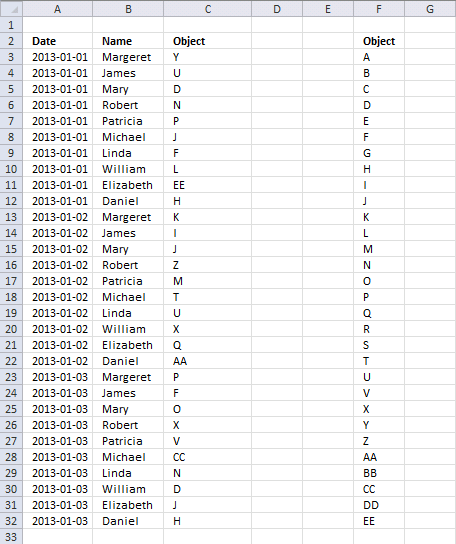Author: Oscar Cronquist Article last updated on April 17, 2013

I've 30 objects and 10 people, and I need to assign each person with randomly (unique) selected objects as a daily activity. Would you please suggest me on how I can do it using excel formulae?The objects are unique within that day. It means that a person can get the same object next day if unlucky. If this is not what you are looking for, let me know.

The array formula in cell C3:C32 returns a random unique value every time you press F9. The objects are in column F.  The table does not need to be sorted by date.

Array formula in cell C3:

=INDEX(\$F\$3:\$F\$32, LARGE((COUNTIFS(\$C\$2:C2, \$F\$3:\$F\$32, \$A\$2:A2,A3)=0)*MATCH(ROW(\$F\$3:\$F\$32), ROW(\$F\$3:\$F\$32)), RANDBETWEEN(1, 31-COUNTIF(\$A\$2:A3, A3))))

How to enter an array formula

1. Select cell C3
2. Copy/Paste above array formula to formula bar
3. Press and hold CTRL + SHIFT
4. Press ENTER

### Explaining array formula in cell C3

Step 1 - Calculate possible objects

COUNTIFS(\$C\$2:C2, \$F\$3:\$F\$32, \$A\$2:A2,A3)=0

becomes

{0; 0; 0; 0; 0; 0; 0; 0; 0; 0; 0; 0; 0; 0; 0; 0; 0; 0; 0; 0; 0; 0; 0; 0; 0; 0; 0; 0; 0; 0}=0

and returns

{TRUE; TRUE; TRUE; TRUE; TRUE; TRUE; TRUE; TRUE; TRUE; TRUE; TRUE; TRUE; TRUE; TRUE; TRUE; TRUE; TRUE; TRUE; TRUE; TRUE; TRUE; TRUE; TRUE; TRUE; TRUE; TRUE; TRUE; TRUE; TRUE; TRUE}

All objects can be randomly selected. If there had been previous object that day they would have been ruled out.

Step 2 - Calculate row numbers

(COUNTIFS(\$C\$2:C2, \$F\$3:\$F\$32, \$A\$2:A2,A3)=0)*MATCH(ROW(\$F\$3:\$F\$32), ROW(\$F\$3:\$F\$32))

becomes

{TRUE; TRUE; TRUE; TRUE; TRUE; TRUE; TRUE; TRUE; TRUE; TRUE; TRUE; TRUE; TRUE; TRUE; TRUE; TRUE; TRUE; TRUE; TRUE; TRUE; TRUE; TRUE; TRUE; TRUE; TRUE; TRUE; TRUE; TRUE; TRUE; TRUE}*{1; 2; 3; 4; 5; 6; 7; 8; 9; 10; 11; 12; 13; 14; 15; 16; 17; 18; 19; 20; 21; 22; 23; 24; 25; 26; 27; 28; 29; 30}

and returns {1; 2; 3; 4; 5; 6; 7; 8; 9; 10; 11; 12; 13; 14; 15; 16; 17; 18; 19; 20; 21; 22; 23; 24; 25; 26; 27; 28; 29; 30}

Step 3 - Return a random number

RANDBETWEEN(1, 31-COUNTIF(\$A\$2:A3, A3))

becomes

RANDBETWEEN(1, 31-1)

becomes

RANDBETWEEN(1, 30)

Step 4 - Return the k-th largest number

LARGE(array, k)

LARGE((COUNTIFS(\$C\$2:C2, \$F\$3:\$F\$32, \$A\$2:A2,A3)=0)*MATCH(ROW(\$F\$3:\$F\$32), ROW(\$F\$3:\$F\$32)), RANDBETWEEN(1, 31-COUNTIF(\$A\$2:A3, A3)))

becomes

LARGE({1; 2; 3; 4; 5; 6; 7; 8; 9; 10; 11; 12; 13; 14; 15; 16; 17; 18; 19; 20; 21; 22; 23; 24; 25; 26; 27; 28; 29; 30}, RANDBETWEEN(1, 30))

and returns a random row number between 1 and 30.

Step 5 - Return a value

=INDEX(\$F\$3:\$F\$32, LARGE((COUNTIFS(\$C\$2:C2, \$F\$3:\$F\$32, \$A\$2:A2,A3)=0)*MATCH(ROW(\$F\$3:\$F\$32), ROW(\$F\$3:\$F\$32)), RANDBETWEEN(1, 31-COUNTIF(\$A\$2:A3, A3))))

becomes

=INDEX(\$F\$3:\$F\$32, random_row_number)

becomes

=INDEX({"A"; "B"; "C"; "D"; "E"; "F"; "G"; "H"; "I"; "J"; "K"; "L"; "M"; "N"; "O"; "P"; "Q"; "R"; "S"; "T"; "U"; "V"; "X"; "Y"; "Z"; "AA"; "BB"; "CC"; "DD"; "EE"}, random_row_number)

and returns a random object.